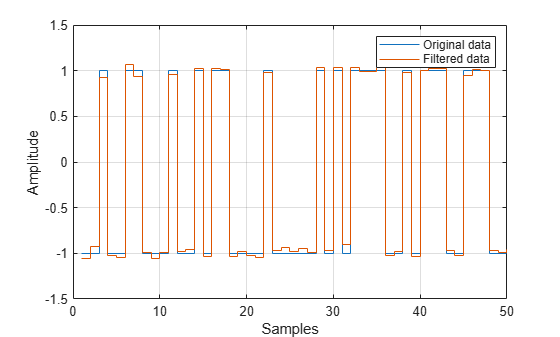Main Content

# comm.IntegrateAndDumpFilter

Integrate discrete-time signal with periodic resets

## Description

The `IntegrateAndDumpFilter` object creates a cumulative sum of the discrete-time input signal, while resetting the sum to zero according to a fixed schedule. When the simulation begins, the object discards the number of samples specified in the Offset property. After this initial period, the object sums the input signal along columns and resets the sum to zero every Ninput samples, set by the integration period property. The reset occurs after the object produces output at that time step.

To integrate discrete-time signals with periodic resets:

1. Define and set up your integrate and dump filter object. See Construction.

2. Call `step` to integrate discrete-time signals according to the properties of `comm.IntegrateAndDumpFilter`. The behavior of `step` is specific to each object in the toolbox.

Note

Starting in R2016b, instead of using the `step` method to perform the operation defined by the System object™, you can call the object with arguments, as if it were a function. For example, ```y = step(obj,x)``` and `y = obj(x)` perform equivalent operations.

## Construction

`H = comm.IntegrateAndDumpFilter` creates an integrate and dump filter System object, `H`. this object integrates over a number of samples in an integration period, and then resets at the end of that period.

`H = comm.IntegrateAndDumpFilter(Name,Value)` creates an integrate and dump filter object, `H`, with each specified property set to the specified value. You can specify additional name-value pair arguments in any order as (`Name1`,`Value1`,...,`NameN`,`ValueN`).

`H = comm.IntegrateAndDumpFilter(PERIOD,Name,Value)` creates an integrate and dump filter object, `H`. This object has the `IntegrationPeriod` property set to `PERIOD` and the other specified properties set to the specified values.

## Properties

 `IntegrationPeriod` Integration period Specify the integration period, in samples, as a positive, integer scalar value greater than `1`. The integration period defines the length of the sample blocks that the object integrates between resets. The default is `8`. `Offset` Number of offset samples Specify a nonnegative, integer vector or scalar specifying the number of input samples that the object discards from each column of input data at the beginning of data processing. Discarding begins when you call the `step` method for the first time. The default is `0`. When you set the `Offset` property to a nonzero value, the object outputs one or more zeros during the initial period while discarding input samples. When you specify this property as a vector of length L, the i-th element of the vector corresponds to the offset for the i-th column of the input data matrix, which has L columns. When you specify this property as a scalar value, the object applies the same offset to each column of the input data matrix. The offset creates a transient effect, rather than a persistent delay. `DecimateOutput` Decimate output Specify whether the `step` method returns intermediate cumulative sum results or decimates intermediate results. The default is `true`. When you set this property to `true`, the `step` method returns one output sample, consisting of the final integration value, for each block of `IntegrationPeriod` input samples. If the inputs are (K$×$`IntegrationPeriod`)$×$L matrices, then the outputs are K$×$L matrices. When you set this property to `false`, the `step` method returns `IntegrationPeriod` output samples, comprising the intermediate cumulative sum values, for each block of `IntegrationPeriod` input samples. In this case, inputs and outputs have the same dimensions.

## Methods

 step Integrate discrete-time signal with periodic resets
Common to All System Objects
`release`

Allow System object property value changes

## Examples

collapse all

Create an integrate and dump filter having an integration period of 20 samples.

`intdump = comm.IntegrateAndDumpFilter(20);`

Generate binary data.

`d = randi([0 1],50,1);`

Upsample the data, and pass it through an AWGN channel.

```x = upsample(d,20); y = awgn(x,25,'measured');```

Pass the noisy data through the filter.

`z = intdump(y);`

Plot the original and filtered data. The integrate and dump filter removes most of the noise effects.

```stairs([d z]) legend('Original Data','Filtered Data') xlabel('Samples') ylabel('Amplitude') grid```expand all

## Algorithms

This object implements the algorithm, inputs, and outputs described on the Integrate and Dump block reference page. The object properties correspond to the block parameters, except:

 The Output intermediate values parameter corresponds to the `DecimateOutput` property.

## Extended Capabilities

Introduced in R2012a

## Support

#### Bridging Wireless Communications Design and Testing with MATLAB

Download white paper